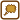## Calculation of Minimal Water Volume

Though we usually suppose that the volume of liquid changes linearly with temperature, this relation is only approximate. More complex relations can describe the phonemenon more precisely.

Let’s suppose that the volume-temperature equation is given by this relation:

V = V0(1 + At + Bt2 + Ct3),

where the temperature is given in Celsius degrees. The constants have been empiricaly found for water in temperature interval between 0 °C and 33 °C:

A = − 6.427·10−5,

B = 8.5053·10−6,

C = − 6.79·10−8.

Determine for which temperature from the given interval is the water’s volume minimal.

• #### Hint

You can use derivation to determine the minimal volume just as you would use it to determine the minimum of a function.

• #### Analysis

The given relation tells us the functional dependence of volume on temperature. We will determine the minimum using the first derivation. The minimum will be located at points where the first derivation equals zero, where it doesn’t exist or in the extreme points of the interval.

We will derivate the function and set equal to zero. This gives us an equation to solve. We will take into consideration only those roots of the equation that are in the interval, the others will be excluded from the solution. We will then calculate the second derivation for roots that are in the interval. If the second derivation is positive then we know that the root is local minimum. We will compare the functional values in the calculated points and the extreme points of the interval. The lowest of the these values will be the minimum of the function on the whole interval.

• #### List of known information

 V = V0(1 + At + Bt2 + Ct3) relation for volume-temperature dependence in temperature interval between 0 °C and 33 °C (temperature is substituted in °C) A = − 6.427·10−5 value of the A constant B = 8.5053·10−6 value of the Bconstant C = − 6.79·10−8 value of the C constant t = ? temperature from the interval for which the volume of water is minimal
• #### Solution

The given relation tells us the volume-temperature functional dependence. We will determine the extreme using derivations:

$\frac{\mathrm{d}V}{\mathrm{d}t}=V_0\left(A+2Bt+3Ct^2\right)\,$

and then we will set the derivation equal to zero:

$\frac{\mathrm{d}V}{\mathrm{d}t}=0,$ $3Ct^2+2Bt+A=0.$

Now we have reached a quadratic equation that has two solutions:

$t_{1{,}2}=\frac{-2B\pm\sqrt{4B^2-12AC}}{6C}\,.$

Substituting for constants A,B and C will give us roots t1 and t2:

$t_{1{,}2}=\frac{-2B\pm\sqrt{4B^2-12AC}}{6C}=$ $=\small \frac{-2\cdot{8.5053}\cdot{10^{-6}}\pm\sqrt{4\cdot\left(8.5053\cdot{10^{-6}}\right)^2-12\cdot{6.427}\cdot{10^{-6}}\cdot{6.79}\cdot{10^{-8}}}}{-6\cdot{6.79}\cdot{10^{-8}}}{\,}^{\circ}\mathrm{C}$ $t_1=3.967\,^{\circ}\mathrm{C}$ $t_2=-79.28\,^{\circ}\mathrm{C}$

The root t2 doesn’t belong to our interval and so we will not take it into consideration. The value of root t1 does belong to the interval but we still have to make sure it is the minimum of the function. We will do so using the second derivation:

$\frac{\mathrm{d^2}V}{\mathrm{d}t^2}=V_0\left(2B+6Ct\right)\,.$

We will substitude in the value of root t1:

$\frac{\mathrm{d^2}V}{\mathrm{d}t^2}\left(t_1\right)=V_0\left(2B+6Ct_1\right)=$ $=V_0\left(2\cdot{8.5053}\cdot{10^{-6}}+{6}\cdot{6.79}\cdot{10^{-8}}\cdot{3.967}\right)=V_0\cdot{1.53}\cdot{10^{-5}}$

We can see that the second derivation is positive in the considered point (volume V0 is definetly positive), which means that the function’s minimum is at this point.

We still have to find out wheather the function reaches the minimum in the extreme points of the interval or not.

We receive this for temperature t = 0 °C:

$V=V_0,$

we receive this for temperature t = 30 °C:

$V=V_0(1+30A+30^2B+30^3C)=1.0039V_0$

and we finally receive this for temperature t = 3.967 °C:

$V=V_0(1+3.967A+3.967^2B+3.967^3C)=0.9999 V_0.$

The minimal volume is reached for temperature 3.967 °C.